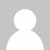Here’s my video with my solutions for the Advent of Code challenge. As usual, let me know in the comments how I could have solved this better.

Here’s my heavy-handed C# and LINQ solution, (drawing on some methods from MoreLINQ)

``````var input = File.ReadAllLines("day5.txt");

var vowels = new[] { 'a', 'e', 'i', 'o', 'u' };
var naughtyStrings = new[] { "ab", "cd", "pq", "xy" };
Predicate<string> hasThreeVowels =
s => s.Where(c => vowels.Any(v => c == v))
.Take(3)
.Count() == 3;
Predicate<string> hasDoubleLetter =
s => s.Pairwise((a, b) => a == b).Any(x => x);
Predicate<string> containsNaughtyString =
s => naughtyStrings.Any(n => s.Contains(n));
Predicate<string> isNice =
s => hasThreeVowels(s) && hasDoubleLetter(s) && !containsNaughtyString(s);

input
.Where(s => isNice(s))
.Count()
.Dump("a"); // a = 236

//"aabcdebccfaa"
Predicate<string> containsNonOverlappingPair = s => s
.Select((c, n) => new { c, n })
.Pairwise((a, b) => new
{
s = new string(new[] { a.c, b.c }),
n = a.n
})
.GroupBy(p => p.s)
.Where(g => g.Count() > 1
&& g.Any(v => v.n - g.First().n > 1))
.Any();

Predicate<string> containsDuplicateSeparatedByOne = s => s
.Select((c, n) => new { c, n })
.GroupBy(p => p.c)
.Where(g => g.Count() > 1
&& g.Pairwise((a,b) => a.n + 2 == b.n).Any(c => c))
.Any();

Predicate<string> isNiceB = s =>
containsNonOverlappingPair(s) && containsDuplicateSeparatedByOne(s);

input
.Where(s => isNiceB(s))
.Count()
.Dump("b"); // b = 51
``````

And here’s a slightly nicer version using Regex (credit to mermop)

``````var input = File.ReadAllLines("day5.txt");
var naughtyStrings = new[] { "ab", "cd", "pq", "xy" };
Predicate<string> hasThreeVowels = s => Regex.IsMatch(s, @"[aeiou].*[aeiou].*[aeiou]");
Predicate<string> hasDoubleLetter = s => Regex.IsMatch(s, @"(\w)\1+");
Predicate<string> containsNaughtyString = s => Regex.IsMatch(s, @"ab|cd|pq|xy");

Predicate<string> isNice =
s => hasThreeVowels(s) && hasDoubleLetter(s) && !containsNaughtyString(s);

input
.Where(s => isNice(s))
.Count()
.Dump("a"); // a = 236

//"aabcdebccfaa"
Predicate<string> containsNonOverlappingPair = s=> Regex.IsMatch(s,@"(\w{2}).*\1+");
Predicate<string> containsDuplicateSeparatedByOne = s => Regex.IsMatch(s,@"(\w).\1");

Predicate<string> isNiceB = s =>
containsNonOverlappingPair(s) && containsDuplicateSeparatedByOne(s);

input
.Where(s => isNiceB(s))
.Count()
.Dump("b"); // b = 51
``````

And finally, the regex solution in F#:

``````let input = File.ReadAllLines("day5.txt")

let (=~) input pattern = Regex.IsMatch(input, pattern)

let hasThreeVowels s = s =~ @"[aeiou].*[aeiou].*[aeiou]"
let hasDoubleLetter s = s =~ @"(\w)\1+"
let containsNaughtyString s = s =~ @"ab|cd|pq|xy"

let isNice s = (hasThreeVowels s) && (hasDoubleLetter s) && (not (containsNaughtyString s))

input
|> Seq.filter isNice
|> Seq.length
|> printf "a: %d"

//"aabcdebccfaa"
let containsNonOverlappingPair s = s =~ @"(\w{2}).*\1+"
let containsDuplicateSeparatedByOne s = s =~ @"(\w).\1"

let isNiceB s =
(containsNonOverlappingPair s) && (containsDuplicateSeparatedByOne s)

input
|> Seq.filter isNiceB
|> Seq.length
|> printf "b: %d"
``````
Want to learn more about LINQ? Be sure to check out my Pluralsight course LINQ Best Practices.``let common predicate =  System.IO.File.ReadAllLines "day05.txt"  |> Array.filter predicate  |> Array.length  // could be Array.sumBy (with 1 or 0 instead of true false in part1 and part2)let (|Matches|) pattern =  let rgx = Regex (pattern, RegexOptions.Compiled)  fun input -> [for m in rgx.Matches input -> [for g in m.Groups -> g.Value]]let part1 () = common (function  Matches   "(?:[aeiou].*){3}" [_]  & Matches "(.)\1"            (_ :: _)  & Matches "ab|cd|pq|xy"      []       -> true| _                                     -> false)let part2 () = common (function  Matches   "(..).*\1" (_ :: _)  & Matches "(.).\1"   (_ :: _) -> true| _                             -> false)``